# Unit conversion + motion problems - math problems

#### Number of problems found: 67

• Cyclist 12What is the average speed of a cycle traveling at 20 km in 60 minutes in km/h?
• Car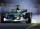Car is going 95 km per hour. How long distance goes in 1 minute?
• RPMAn electric motor makes 3,000 revolutions per minutes. How many degrees does it rotate in one second?
• RunningJoanne ran 8 1/2 Kyleigh 1 2/3 and John 6 3/4 miles in one day. How many miles did the girls run in 3 days?
• Motion problemFrom Levíc to Košíc go car at speed 81 km/h. From Košíc to Levíc go another car at speed 69 km/h. How many minutes before the meeting will be cars 27 km away?
• Car loop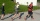The toy car runs at an average speed of 2 m/s. In a minute, it will pass the entire circuit five times. How long is the loop?
• Avg speed of flight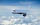The students vice adventure had a 2,367 km flight. If they travel time was 2 hours and 56 minutes, what was their average speed in kilometres per hour?
• Two trains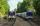Two trains go at each other. First at speed 40 km/h and the second at speed 60 km/h. At what distance will be 30 minutes before they will meet?
• Passenger car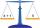Passenger car travels 1 km for 2.3 minutes, a truck during a 50 seconds long. How much is the speed of the truck less?
• Earth and Sun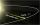Earth is 150 million km away from the Sun, 300,000 km of sunlight travels in 1 second. How long will the light come from the Sun to the Earth?
• The train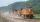The train passed the observer in the 20s and passed through a 1500m tunnel in 2 minutes. Calculate the speed (km/h) and train length.
• Runner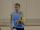Peter ran a speed of 12.4 km / h. After 45 minutes running he had a hiatus. The track is 31 km long. How many kilometers he still to run if he still running at the same speed?
• Two busesThe first bus runs 15 minutes the second bus runs after 21 minutes. Together they both leave at 7:00 on Monday. When and what day will they meet?
• Two cars 2Two cars started from two positions 87 km distant at the same time in opposite directions at speeds 81 km/h and 75 km/h. What was the distance between them after 2 hours 50 minutes of driving.
• Two shipsThe distance from A to B is 300km. At 7 am started from A to B a ferry with speed higher by 20 km/h than a ship that leaves at 8 o'clock from B to A. Both met at 10h 24min. Determine how far they will meet from A and when they reach the destination.From the junction of two streets that are perpendicular to each other, two cyclists (each on another street) walked out. One ran 18 km/h and the second 24 km/h. How are they away from a) 6 minutes, b) 15 minutes?The railroad runs parallel to the railway. The train travels at 36 km/h on track. In the opposite direction, the motorcycle rides at 90 km/h. The train passes in 3 seconds. How long is a train?
• Average speed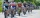The average speed of a pedestrian who walked 10 km was 5km/h, the average speed of a cyclist on the same track was 20km/h. In how many minutes did the route take more than a cyclist? Q
• Two trainsThe train runs at speed v1 = 72 km/h. The passenger, sitting in the train, observed that a train long l = 75m in 3 s passed on the other track in the opposite direction. Calculate the speed of this train.
• Train and walker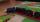Walker passes an hour 5 km. Express train per hour 100 km. Walker went 4 hours. How many minutes took the distance express train?

Do you have an interesting mathematical word problem that you can't solve it? Submit a math problem, and we can try to solve it.

We will send a solution to your e-mail address. Solved examples are also published here. Please enter the e-mail correctly and check whether you don't have a full mailbox.

Please do not submit problems from current active competitions such as Mathematical Olympiad, correspondence seminars etc...